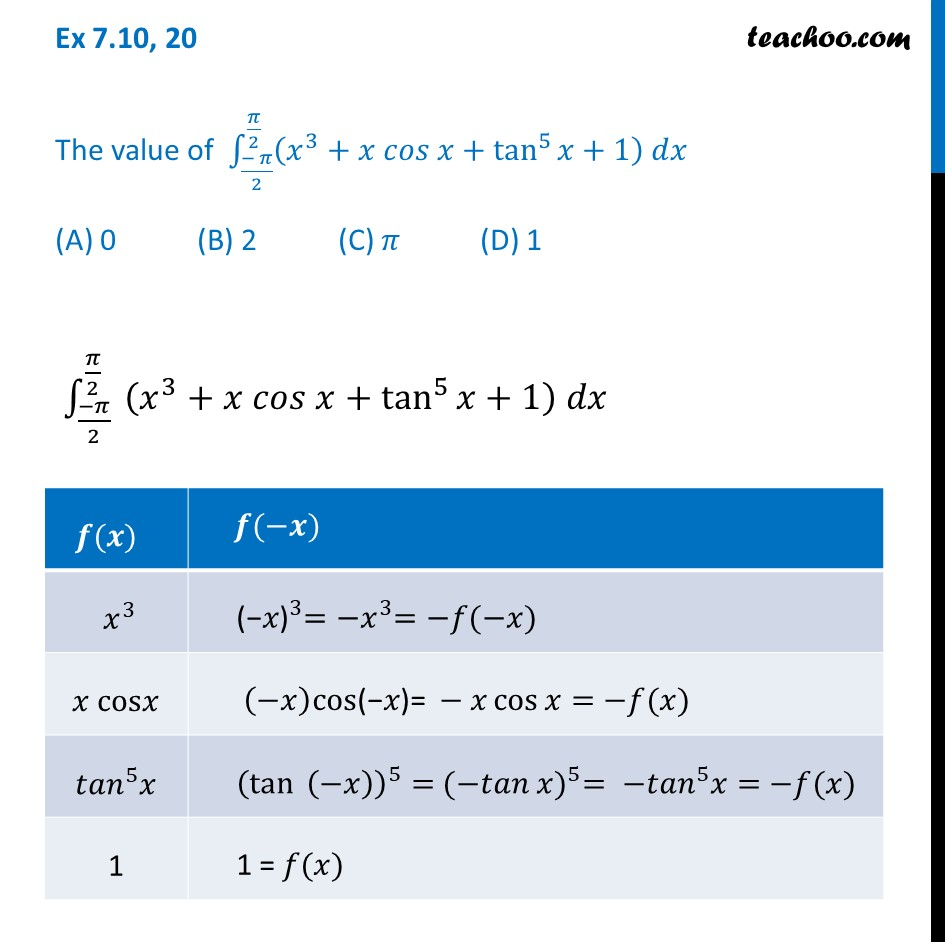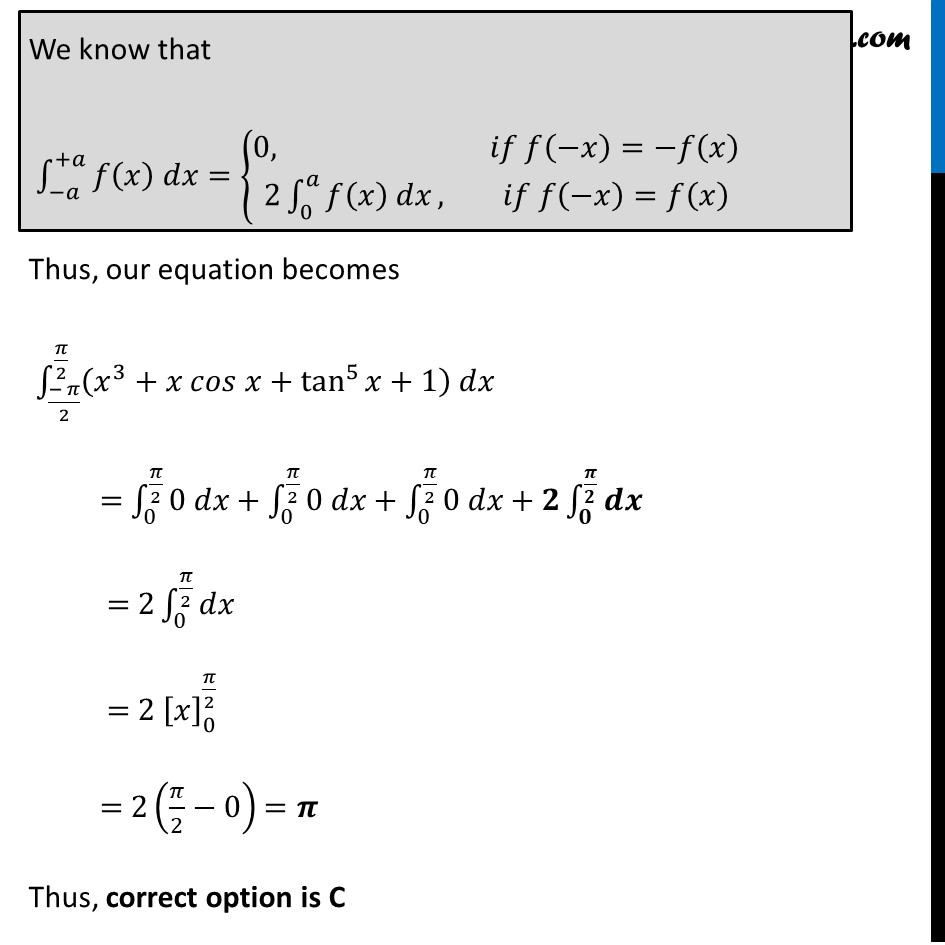Ex 7.10

Chapter 7 Class 12 Integrals
Serial order wiseLearn in your speed, with individual attention - Teachoo Maths 1-on-1 Class

### Transcript

Ex 7.10, 20 The value of ∫_((− 𝜋)/2)^(𝜋/2)▒〖(𝑥^3+𝑥 𝑐𝑜𝑠 𝑥+tan^5⁡〖𝑥+1〗 ) 𝑑𝑥〗 (A) 0 (B) 2 (C) 𝜋 (D) 1 ∫_((−𝜋)/2)^(𝜋/2)▒〖 (𝑥^3+𝑥 𝑐𝑜𝑠 𝑥+tan^5⁡〖𝑥+1〗 ) 𝑑𝑥〗 Thus, our equation becomes ∫_((− 𝜋)/2)^(𝜋/2)▒〖(𝑥^3+𝑥 𝑐𝑜𝑠 𝑥+tan^5⁡〖𝑥+1〗 ) 𝑑𝑥〗 = ∫_0^(𝜋/2)▒〖0 𝑑𝑥+∫_0^(𝜋/2)▒〖0 𝑑𝑥+∫_0^(𝜋/2)▒〖0 𝑑𝑥+𝟐∫_𝟎^(𝝅/𝟐)▒𝒅𝒙〗〗〗 = 2∫_0^(𝜋/2)▒𝑑𝑥 = 2 [𝑥]_0^(𝜋/2) = 2(𝜋/2−0) =𝝅 Thus, correct option is C